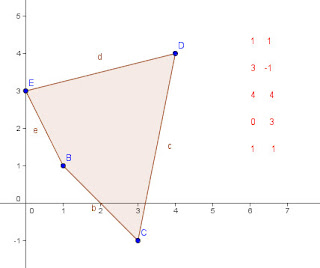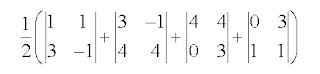## Sunday 5 January 2020

### The Shoelace Formula and a Formulas for ShoelacesThe shoelace formula is an algorithm for finding the area of a polygon in the plane when the coordinates (x,y) are known.For example if the coordinates of a quadrilateral are given as (1,1); (3, -1); (4,4); and (0,3) then the area can be calculated by putting these in two columns (or rows as shown below) and multiplying along diagonals as shown (notice that the first coordinate pair are repeated at the end for ease of computation).The diagonal products to each side are summed, then the absolute value of the difference of the two sums is multiplied by 1/2 to give the area. In this case the right products total to 23, the left to 2, for a difference of 21, so the area is one-half of that, or 10.5 square units. The points have to be taken in order around the polygon in either clockwise or counter-clockwise order using each number once as the first pair. It is the criss-cross appearance of the diagonals that draws the "shoestring" name to the apporach.

The shoelace formula is just a simple diagrammatic extension of an earlier determinant method sometimes (perhaps seldom?) called the Surveyor's formula (not to be confused with the Ptolemaic Egyptian method of the same name for finding areas of quadrilaterals), which does the same computation with a sequence of determinants, like this: Note thatThis gives ½(-4+16+12-3) which is again the 10.5 sq units we had before. The calculation of each determinant constitute one product from each side of the shoelace algorithm and calculates their difference. (the sum of the differences is the same as the difference of the sums).

Additional illustrations and worked examples can be found at this file by Charles L. Hamberg and Ronald Vavrinek, from the Illinois Mathematics and Science Academy. It also includes a program you can use on your ti-84 or other programmable calculator.

While we are talking about shoelaces, they also showed up in an interesting book by Australian Mathematician Burkard Polster. In "The Shoelace Book", Polster examines various approaches to methods of lacing a pair of shoes, and "mathematizes" lacings formally enough to enumerate the possible lacing paths.. For a shoe with six eyelets on each side there turns out to be 43,200 different paths for a shoelace to pass through every eyelet, even with the added condition that each eyelet must contribute to the essential purpose of pulling the two halves of the shoe together..... go ahead, take a minute and check...I'll wait... dum de dumm..de dumm (all done?) OK.. If that was more difficult than you might have expected, you can find a good link that gives a glimpse of the work at Google Books where he describes lacings by such names as Crisscross, zigzag, bowtie, devil, angel, and star... I'm thinking of switching to the bowtie lacing myself, not just because it is efficient, but I like the look.

And in case you think this is all trivial math, take a look at this page from the early pages of the book:

You can also order the book from Amazon

Speaking of strings (ok, that's a stretch from the "shoestring" idea) this is blog number 300 on Blogger.... I think I'm a veteran...

(I'm actually reposting this updated post as blog number 3691, fingers crossed, I may update it again as blog 5xxx.)